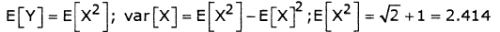Courses

# Test: Digital Communications - 1

## 15 Questions MCQ Test GATE ECE (Electronics) 2022 Mock Test Series | Test: Digital Communications - 1

Description
This mock test of Test: Digital Communications - 1 for Electronics and Communication Engineering (ECE) helps you for every Electronics and Communication Engineering (ECE) entrance exam. This contains 15 Multiple Choice Questions for Electronics and Communication Engineering (ECE) Test: Digital Communications - 1 (mcq) to study with solutions a complete question bank. The solved questions answers in this Test: Digital Communications - 1 quiz give you a good mix of easy questions and tough questions. Electronics and Communication Engineering (ECE) students definitely take this Test: Digital Communications - 1 exercise for a better result in the exam. You can find other Test: Digital Communications - 1 extra questions, long questions & short questions for Electronics and Communication Engineering (ECE) on EduRev as well by searching above.
QUESTION: 1

###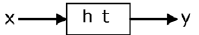has P.S.D. Sxx f then y has P.S.D

Solution: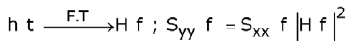QUESTION: 2

### Consider Y= ax+b, a>0. If the density and distribution functions of x are F. (x) and fx (x), then Fy (y) is equal to

Solution: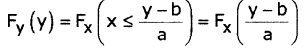QUESTION: 3

### Consider a random process x(t). Which of the following can represent Rx. (r)?

Solution: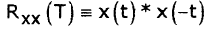*Answer can only contain numeric values
QUESTION: 4

It is required to transmit at 100 Kbit/s and a bandwidth of 2 MHz is used. The

minimum S/N ratio is ___________________

Solution: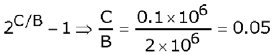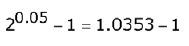*Answer can only contain numeric values
QUESTION: 5

SNR when the system is uncoded is 4 dB, when it is coded is 2 dB then the coding gain is ________________  (dB)

Solution:

Coding gain =  SNR encoded - SNR. coded = 4.dB-2dB = 2dB

QUESTION: 6

In the set up shown below, determine the number of bits required to encode each sample of x (t) such that bandwidth of signal y(t) is greater than 5 MHz.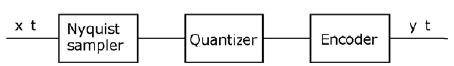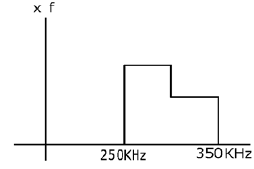Solution:

Nyquist sampler is a sampler that samples a given signal at a rate twice the highest frequencies

fs = 2 fm, f5 = 2 x 350 kHz = 0.7MHz

Band width of output of PCM =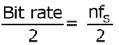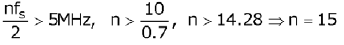QUESTION: 7

A sinusoidal signal whose peak-peak amplitude is 6 V is modulated using deltamodulator. At what rate should be sinusoidal signal sampled such that slope over load distortion is prevented with step size of 0.942 V?

Solution:

2A = 6V ⇒ A = 3V

In order to overcome slope over load distortion in delta modulator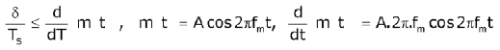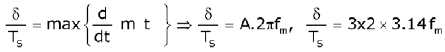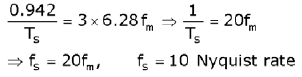QUESTION: 8

The probability of the five possible outcomes of an experiment are given as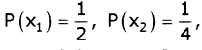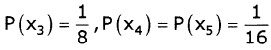Determine the informationrate, if there are 8 outcomes per second.

Solution: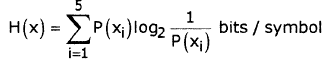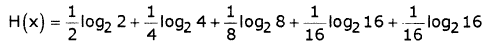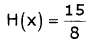= 1.875 bits / sec; r=8 outcomes/sec
Information rate R=r.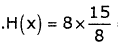=15bits / sec

QUESTION: 9

Probability of picking 3 symbols are P0, P1 and P2 respectively. The maximum entropy is achieved, when P0, P1, P2 are

Solution:

PO =P1 =P2 =P; PO + P1 + P2 = 1; 3P=1 ⇒ P= 1/3

QUESTION: 10

Hartley's law can be defined as r = 2 Blog2 M . When compared to Shannon Hartley theorem, C, M are

Solution:

Comparing C=B log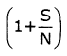; r = C;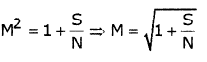QUESTION: 11

The probability of a bit decoded correctly at receiver is `p'. If n bits are sent, what is the probability that one bit is decoded correctly?

Solution:

correct : p; error: 1-p

out of n bits, only one bit is decoded correctly=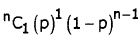QUESTION: 12

A stationary random process x(t) has the following correlation function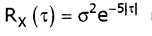is constant) . Find the psd of random signal x(t).

Solution:

PSD of input is expressed as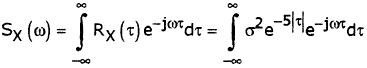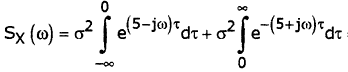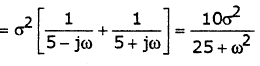QUESTION: 13

If random variable 'x' has Gaussian pdf with mean M, then y=2x has PDF andmean respectively

Solution: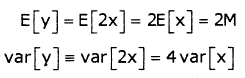so y has pdf N(2m,402) which is Gaussian

QUESTION: 14

If x has P.S.D Sxx (f), then y has P.S.D of ( Assume H(f) is the Fourier Transform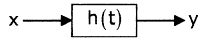Solution: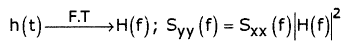QUESTION: 15

A Gaussian random variable 'x' has mean 1 and variance√2. If y = x2, then the mean of y is

Solution: